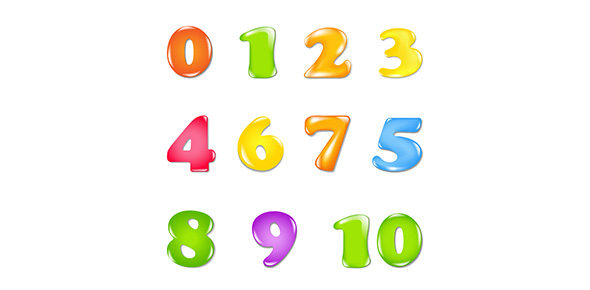# Exam 7 Preap Numbers 1-5

5 Questions | Total Attempts: 147Settings.

• 1.
According to Hooke’s law, the force exerted by a spring on an object is proportional to
• A.

The mass of the object.

• B.

The displacement of the spring.

• C.

The length of the spring.

• D.

The volume of the object.

• 2.
In any system in simple harmonic motion, the restoring force acting on the mass in the system is proportional to
• A.

Displacement.

• B.

The length of a pendulum.

• C.

The mass.

• D.

Frequency.

• 3.
The spring constant in a given oscillating mass-spring may be changed by
• A.

Increasing the mass.

• B.

Decreasing the mass.

• C.

Decreasing the initial displacement.

• D.

D. none of the above d. none of the above none of these answers

• 4.
In an oscillating mass-spring system, the velocity of the mass is greatest when the mass is
• A.

At the point of maximum displacement.

• B.

Halfway between the equilibrium point and maximum displacement.

• C.

At the point where acceleration is greatest.

• D.

At the equilibrium point.

• 5.
As the swinging bob of a pendulum moves farther from its equilibrium position, the pendulum’s _______________ increases.
• A.

Frequency

• B.

Mass

• C.

Restoring force

• D.

Length

Related TopicsBack to top